# Top 30 Microsoft Excel Interview Questions

Q11. Is it possible to make Pivot Table using multiple sources of data? How?

Answer: Yes, this is possible by using data modelling technique.

• Import from a relational database, like Microsoft SQL Server, Oracle, or Microsoft Access. You can import multiple tables at the same time.
• Import multiple tables from other data sources including text files, data feeds, Excel worksheet data, and more. You can add these tables to the Data Model in Excel, create relationships between them, and then use the Data Model to create your PivotTable.

How to use Data Modeling for creating Pivot Table:-

After creating relationships between tables, make use of the data for analysis.

• Click any cell on the worksheet
• Click Insert > PivotTable
• In the Create PivotTable dialog box, under Choose the data that you want to analyze, click Use an external data source
• Click Choose Connection.
• On the Tables tab, in This Workbook Data Model, select Tables in Workbook Data Model.
• Click Open, and then click OK to show a Field List containing all the tables in the Data Model.

Q12. What is Ribbon in MS-Excel?

Answer: The ribbon in Excel consists of the tabs at the top. These tabs are split into groups which categorize related command buttons into sub tasks.

Each group has its respective command button and the dialog box launcher, which are present in the lower right corner in some of the groups.

This opens a dialog box containing a bunch of additional options we can choose from.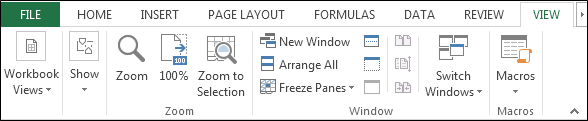As per Excel’s default settings, we have 8 tabs. Which are:

• File
• Home
• Insert
• Page Layout
• Formulas
• Data
• Review
• View

To know more in detail click here

Q13. Which option do we use to adjust the text within a cell and what is the procedure to do it?

Answer: To adjust text in a cell, we use Wrap text option. It can be used in two ways:

Option 1: In the Home tab > Alignment > Wrap Text.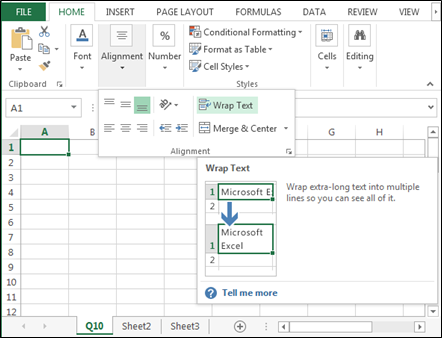Option 2:

• Press Ctrl+1 on your keyboard
• Format cells dialog box will appear
• In the Alignment Tab
• Click on Wrap text
• And then click on OK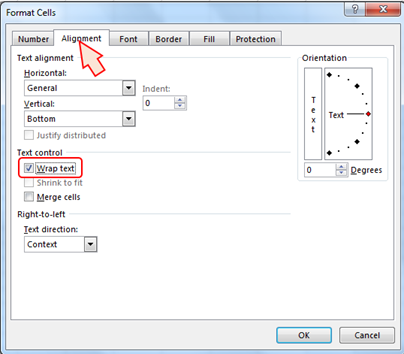Check for more examples:

1. #### Automatically & Manually Wrapping text

Q14. How to select all the objects in the sheet?

Answer: To select the object, we use Go to Special option.

Follow the below steps to select the objects:

• Press the shortcut key F5 to open the Go to Special dialog box
• Click on Special > Click on object > Click on OK
• All objects will get selected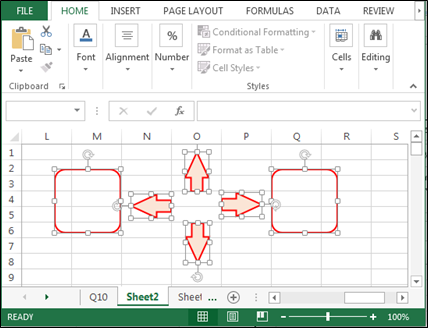Q15. What is IF function in Microsoft Excel?

Answer:  ‘If function’ is one of the logical functions in Excel. We use this function to check the logical condition and specify the value whether it’s true or false. ‘If function’ has three arguments but only first argument is mandatory and other two are optional.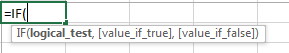Q16. What is the use of Name box?

Answer:  Name Box is located in the left most corner of the Excel sheet. Usually, we use Name box to check the cell reference to the active cell but it has several other uses too.

For Example: We can define the name of the range through Name box. Below are the steps to understand this statement:

• Select the range
• Edit in the Name box
• Type Weeks > Press Enter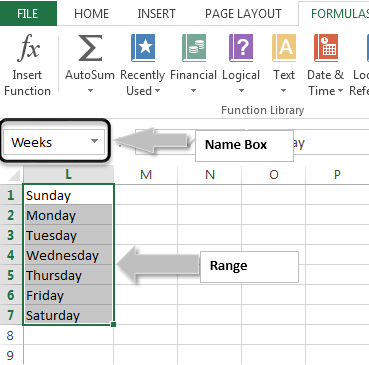Q17. What is the use of Vlookup and how do we use it?

Answer:  Vlookup is used to find the data in the large spreadsheet by lookup value in another worksheet. To use the Vlookup function, we should have common values in both data. For example, we want to search the phone number of a person. So, in order to find out the phone number, we will need the concerned person’s name.

How do we use it?

We have 2 set of HR data in Excel. In the second data, we want to update joining date of every employee from the first data. To use the Vlookup function, data must have the common value.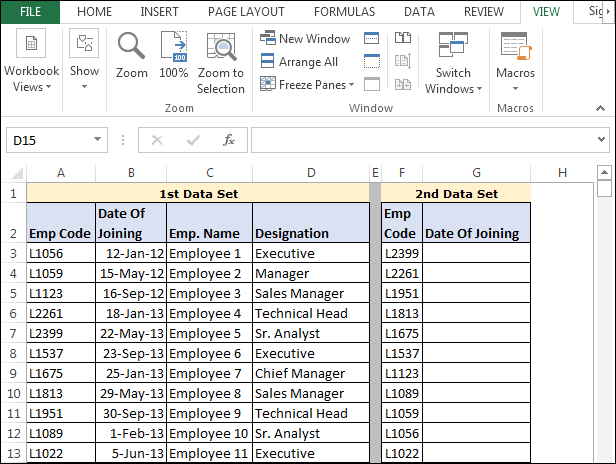• Enter the formula in cell G3
• =VLOOKUP(F3,\$A\$3:\$D\$13,2,0)
• Press enter and copy the same formula in the range F4:F13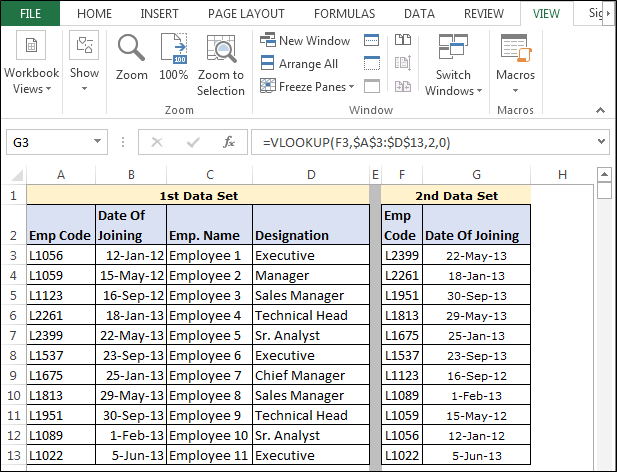Formula Explanation: =VLOOKUP(F3,\$A\$3:\$D\$13,2,0)

• In this formula, F3 is the cell of common value or lookup value
• Then we have selected the range \$A\$3:\$D\$13 to the 1st data
• 2: we have defined to pick the value from the 2nd column
• 0: we have defined for the exact match

Q18. How can we view the values in the right most column in Excel?

Answer:  We can view the value from the right most column through Index and Match function.

Example: We have 2 HR data in Excel. In the second data, we want to update joining date of every employee, from the first data. To use the Vlookup function, data must have the common value.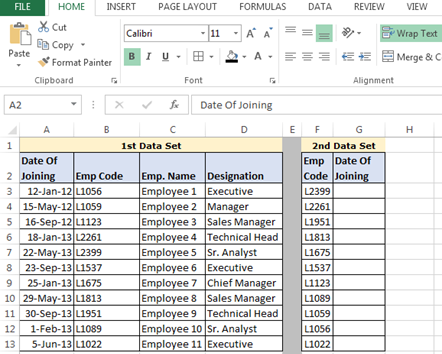• Enter the formula in cell G3
• =INDEX(\$A\$3:\$D\$13,MATCH(F3,\$B\$3:\$B\$13,0),1)
• Press Enter
• Copy the formula in range G4:G13.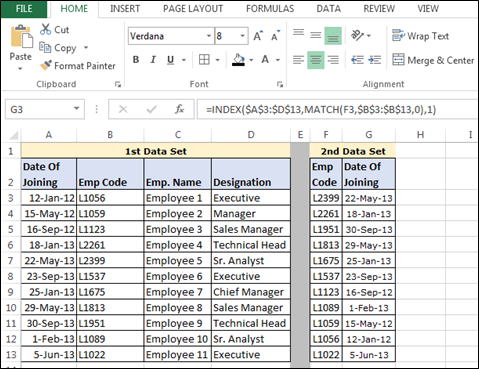Formula Explanation: =INDEX(\$A\$3:\$D\$13,MATCH(F3,\$B\$3:\$B\$13,0),1)

• In this formula =INDEX(\$A\$3:\$D\$13 this syntax is used to define the array from which we want to pick the value
• MATCH(F3,\$B\$3:\$B\$13,0) this syntax will help to lookup the value
• At last ‘1 define’ is to pick the value as result so 1 implies that we want to pick the value from the 1st column

Q19. How can we merge multiple cells text strings in a cell?

Answer: We can merge multiple cells text string by using the Concatenate function and “&” function.

Example: We have three names: First Name, Middle name, Last name in 3 columns. To merge the names and make it a full name, follow the steps below: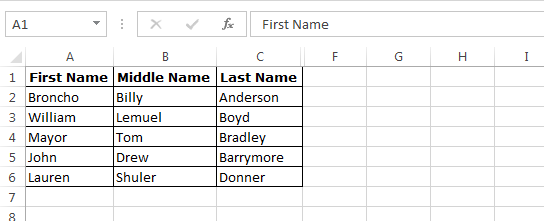Concatenate Function

• Enter the formula in cell D2
• =CONCATENATE(A2," ",B2," ",C2)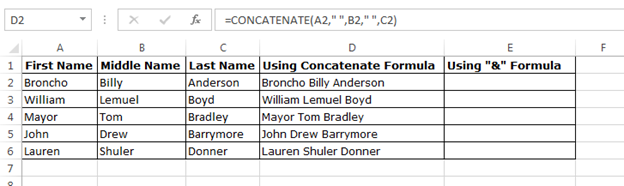“&” use in formula to merge the text:

• Enter the formula in cell E2
• =A2&" "&B2&" "&C2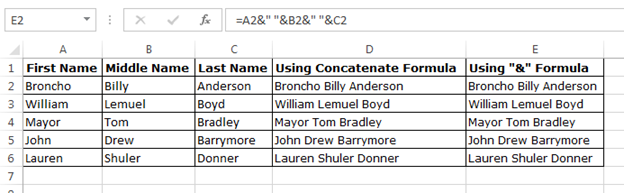Q20. What is Sumif function and how to use it?

Answer: We use Sumif function to add the cells specified by a given condition or criterion.

Syntax Range Criteria Sum_Range
=SUMIF(range, criteria,[sum_range]) Data range from which we want to retrieve the sum For which we want to calculate the sum from the data The range of column from which we want calculate the sum

How to use it?

We have HR data in which we have salary details of every employee, department wise. Now, we want to retrieve the total salary amount department wise.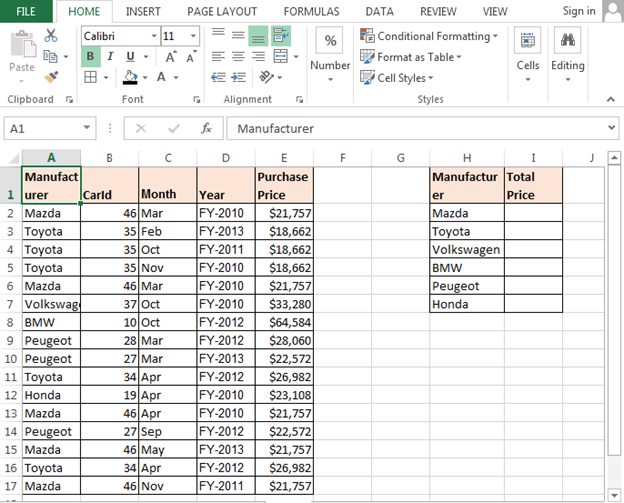• Enter the formula in cell I2
• =SUMIF(\$A\$2:\$E\$17,\$H2,\$E\$2:\$E\$17) and press Enter
• Copy the same formula in the range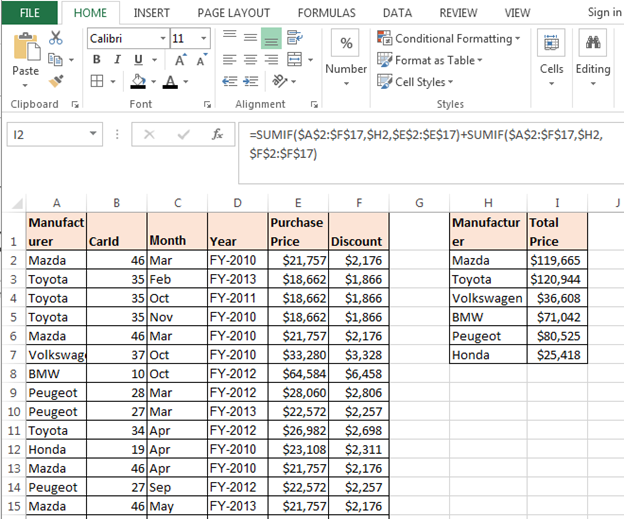Formula Explanation:

• \$A\$2:\$E\$17it is the range of data
• \$H2 is the criterion for which formula will calculate the sum
• ,\$E\$2:\$E\$17is the sum range in the data

We would love to hear from you, do let us know how we can improve, complement or innovate our work and make
it better for you. Write us at info@exceltip.com

## Users are saying about us...

1.SUMIF is so 2003

2.Excellent Boss. Thanks & Great Job

3.4.for Q18. the forumla is wrong :
Formula Explanation: =INDEX(\$A\$3:\$D\$13,MATCH(F3,\$B\$3:\$B\$13,0),1)
the match function should have lookuparray as \$A2:\$D2 instead.

5.Hi All Geniuses,

Please assist me on below query using pivot table.

I want to use % of Room revenue from Total Revenue for all 3 months.

I will use formula (Room revenue / Total Reveue)

1 month: (1000 / 10000 = 10%)
2 month: (800 / 12000 = 7%)
3 month: (1000 / 8000 = 13%)

I want the same formula to be apply for grand total. (2800 / 30000 = 9%)

But it is wrongly showing as Total of all 30%.